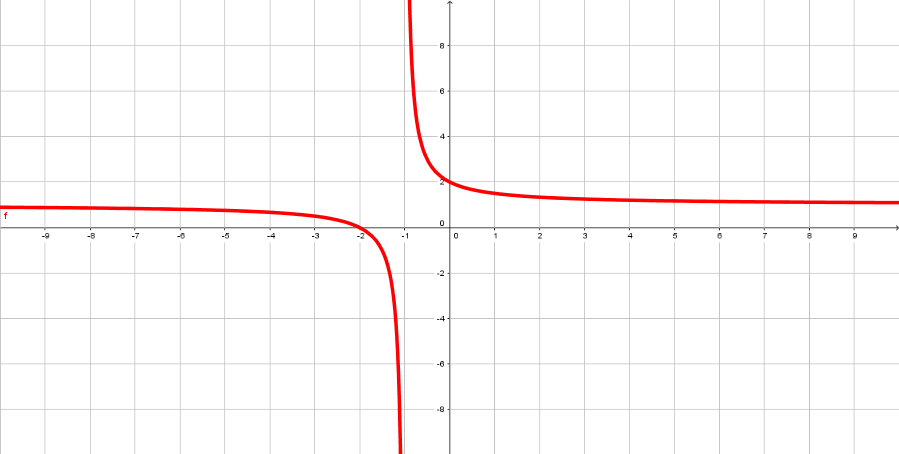# 005 Sample Final A, Question 20

Question Consider the following rational function,

$f(x)={\frac {x^{2}+x-2}{x^{2}-1}}$a. What is the domain of f?
b. What are the x and y-intercepts of f?
c. What are the vertical and horizontal asymptotes of f, if any? Does f have any holes?
d. Graph f(x). Make sure to include the information you found above.

Foundations:
1) What points are not in the domain of f(x)?
2) How do you find the intercepts?
3) How do you find the asymptotes and zeros?
4) How do you determine if f has any holes?
1) The point that are not in the domain of f(x) are zeros of the denominator.
2) To find the x-intercepts set y = 0 and solve for x. For y-intercepts set x = 0 and simplify.
3) For zeros, find the zeros of the numerator. Vertical asymptotes correspond to zeros of the denominator. Horizontal asymptotes correspond to taking the limit as x goes to $\infty$4) Holes occur when a single value of x is a zero of both the numerator and denominator.

Solution:

Step 1:
We start by finding the zeros of the denominator since this will give us information about vertical asymptotes and the domain. The zeros of the denominator are x = -1, 1. This tells us the domain is $(-\infty ,-1)\cup (-1,1)\cup (1,\infty )$and the potential vertical asymptotes are x = -1 and x = 1.
Step 2:
Now we should find the y-intercepts(zeros) to determine if f has any holes, which are zeros of numerator and denominator. Thus, y-intercepts correspond to zeros of the numerator x = -2, 1. Now we know we have a hole at x = 1, and a y-intercept at $(-2,0).$Step 3:
For the horizontal asymptote take the limit as x goes to $\infty .$This tells us that the horizontal asymptote is y = 1.
Domain: $(-\infty ,-1)\cup (-1,1)\cup (1,\infty )$The x-intercept is $(-2,0)$and y-intercept is $(0,2)$